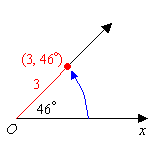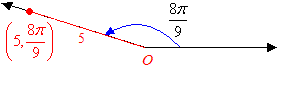# Polar Coordinates

In a plane, suppose you have a point $O$ called the origin, and an axis through that point – say the $x$ -axis – called the polar axis.

Then the polar coordinates $\left(r,\theta \right)$ describe the point lying a distance of $r$ units away from the origin, at an angle of $\theta$ to the $x$ -axis. The value of $\theta$ may be given in degrees or radians .

Examples:To convert from polar coordinates to Cartesian coordinates, you can use:

$\begin{array}{l}x=r\mathrm{cos}\theta \\ y=r\mathrm{sin}\theta \end{array}$

To convert from Cartesian coordinates to polar coordinates:

$r=\sqrt{{x}^{2}+{y}^{2}}$ .

Since $\mathrm{tan}\theta =\frac{y}{x},\text{\hspace{0.17em}}\text{\hspace{0.17em}}\text{\hspace{0.17em}}\theta ={\mathrm{tan}}^{-1}\left(\frac{y}{x}\right)$ .

So, the Cartesian ordered pair $\left(x,y\right)$ converts to the Polar ordered pair $\left(r,\theta \right)=\left(\sqrt{{x}^{2}+{y}^{2}},{\mathrm{tan}}^{-1}\left(\frac{y}{x}\right)\right)$ .